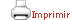Teaching plan for the course unit

(Short version)

 Català English CloseGeneral information

Course unit name: Linear Models

Course unit code: 361231

Coordinator: Francisco De Asis Carmona Pontaque

Department: Department of Genetics, Microbiology and Statistics

Credits: 6

Single program: S

 Estimated learning time Total number of hours 150

 Face-to-face and/or online activities 60
 -  Lecture with practical component Face-to-face and online 15 -  Problem-solving class Face-to-face and online 22,5 -  IT-based class Face-to-face and online 22,5
 Supervised project 40
 Independent learning 50

 Learning objectives
 Referring to knowledge The main objective of the course is to understand linear models and their application to the most common situations: — Know the processes of parameter estimation in a linear model. — Know the decision-making mechanisms associated with the main hypothesis tests in linear models. — Know how to characterize a simple and multiple linear regression model. — Know how to validate linear regression models. — Know how to characterize some simple models of variance analysis.  Referring to abilities, skills — Know how to solve the parameter estimation of a linear model. — Know how to analyse the main hypothesis tests in linear models. — Know how to validate a linear regression model. — Know how to validate a linear regression model. — Know how to solve some simple models of variance analysis. — Be able to interpret the results rigorously.

 Teaching blocks

1. Simple linear regression

1.1. Estimation of regression coefficients by least squares

1.2. Decomposition of variability

1.3. Correlation coefficient and determination coefficient

1.4. Inference on regression parameters

1.5. Prediction

1.6. Matrix approach

2. Regression models

2.1. Multiple linear regression

2.2. Measures of best fit

2.3. Inference on regression coefficients

2.4. Standardized regression coefficients

2.5. Polynomial regression

2.6. Introduction to model diagnosis

2.7. Variable selection

2.8. Robust regression

2.9. Simultaneous coefficient penalization

2.10. Logistic regression

3. The linear model

3.1. Estimation of parameters by least squares

3.2. Properties of estimators

3.3. Linear hypothesis testing

3.4. Model testing

3.5. Estimable parametric functions

4. Linear model of variance analysis

4.1. Single factor model

4.2. Comparison of means

4.3. Other models

4.4. Introduction to covariance analysis

Book

CARMONA, Francesc. Modelos lineales. Barcelona: Publicacions i Edicions de la Universitat de Barcelona, 2005

 A good book on the subject of linear models, but note that it is more advanced in terms of content than is required for this course.

FARAWAY, Julian James. Linear Models with R. Chapman & Hall/CRC Press, 2014

FOX, J and WEISBERG, S. An R Companion to Applied Regression. SAGE Publications; Inc, 2018.

 This book covers one part of the course.

RAWLINGS, John O. Applied Regression Analysis: a research tool. New York [etc.]: Springer, 1998

 This book, in English, is highly recommended for the examples it offers and their corresponding commentaries.

MONTGOMERY, Douglas C. et al. Introduction to Linear Regression Analysis. 2nd ed. New York [etc.]: Wiley, 1992

OLIVA, Francesc, et al. Propietats i eines d’àlgebra matricial per a estadística. Barcelona : Universitat de Barcelona, 1995

Web page

The R Project for Statistical Computing